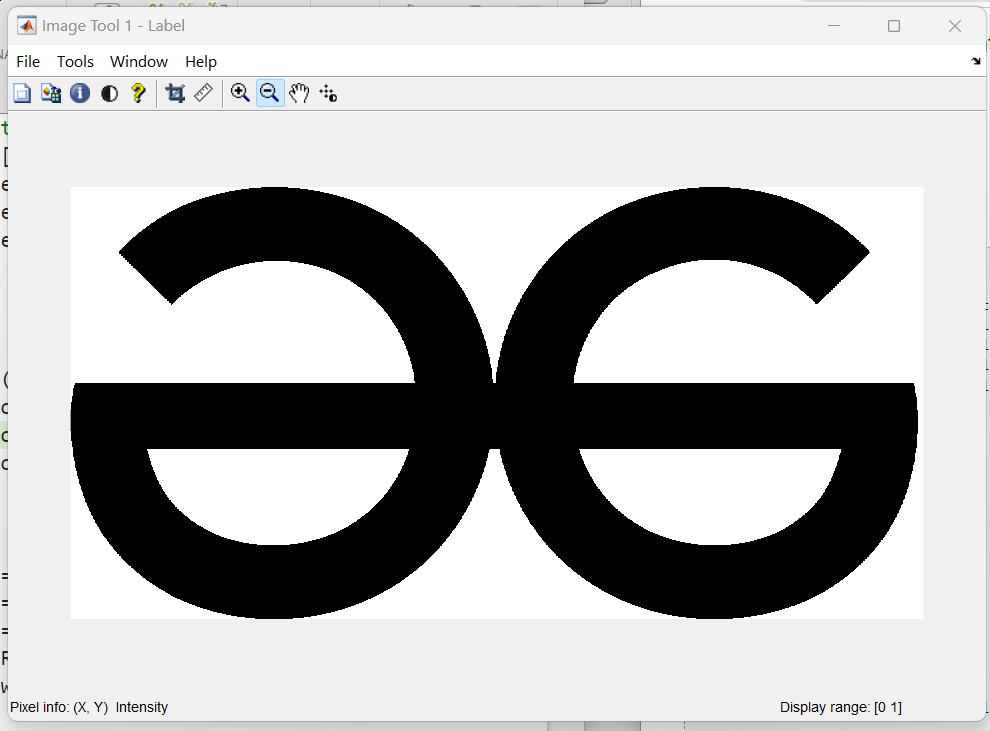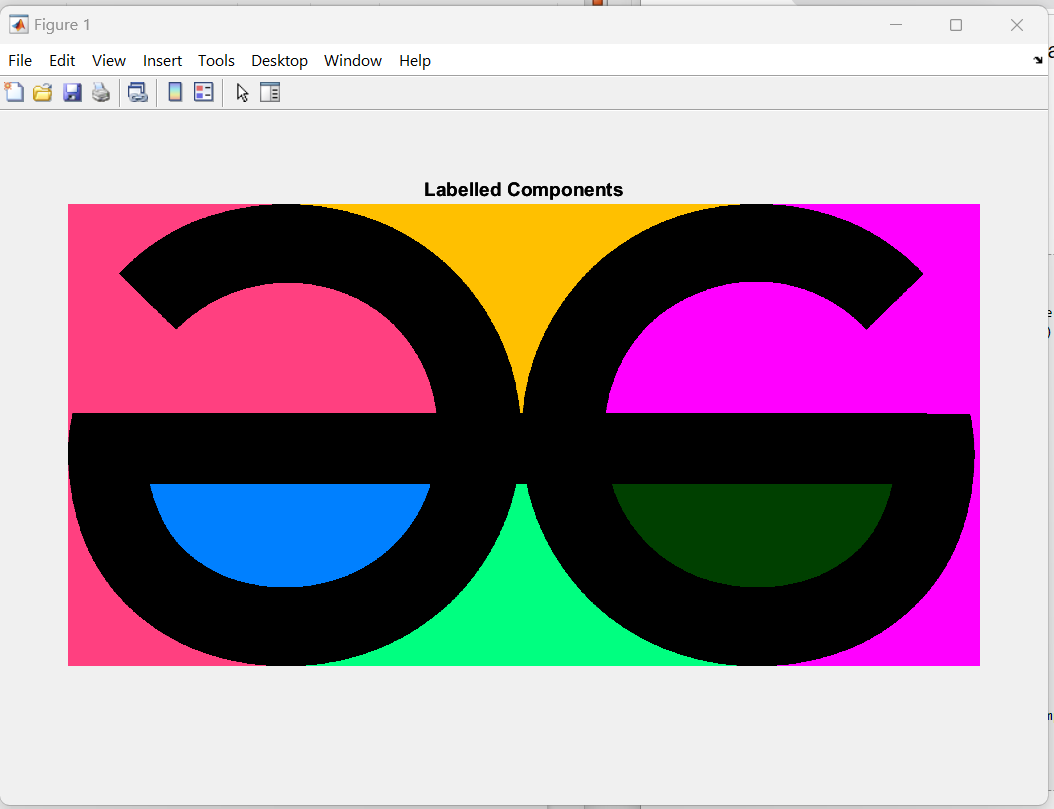GFG App
Open AppBrowser
Continue

# MATLAB: Connected Component Labeling without Using bwlabel or bwconncomp Functions

A connected component or object in a binary image is a set of adjacent pixels. Determining which pixels are adjacent depends on how pixel connectivity is defined. There are two standard classification connections for 2D images.

1. Connected-4 – Pixels are connected when the edges touch. Two adjacent pixels are part of the same object if they are both on and connected along the horizontal or vertical direction.
2. Connected-8 – Pixels are connected when their edges or corners touch. Two adjacent pixels are part of the same object if they are both on and connected along a horizontal, vertical, or diagonal direction.

So in this article we will understand the Connected Component labeling without using bwlabel or bwconncomp functions, before performing the labeling we have to understand the basic required terms-

### Labeling

labeling means is to identify and placing labels on each program of an object project in the image. in Binary image, it’s classified as 4-connected and 8-connected. for performing the labeling in MATLAB we use the Built-in function bwlabel() used to label the object in the binary image.

MATLAB has a function called bwlabel for labeling connected components. Connected components are extracted based on the iterative formula:

Xk   =  ( Xk-1  ⊕  B ) ∩ A     where k = 1,2,3 …..

### Implementation

To perform Connected Component labeling without using bwlabel or bwconncomp functions:

If we use the built-in function bwlabel or bwconncomp to perform the labeling it is easier than without using these functions, but here the MATLAB code may be a little bit longer.

Here we use the given GeeksforGeeks logo to perform the labeling without using the built-in function. So firstly we have to plot the image and convert it into a binary image, then define the structure of the image to initialize the Label matrix with zero.

Example 1:

## Matlab

 `I=imread(``'GFG.png'``); ` `I=im2bw(I); ` ` `  `%Structuring element ` `B=strel(``'square'``,3); ` `A=I; ` ` `  `% Find a non-zero element's position. ` `p=find(A==1); ` `p=p(1); ` `Label=zeros([size(A,1) size(A,2)]); ` `N=0; ` `while``(~isempty(p)) ` `    ``N=N+1; ` `     `  `    ``%Label for each component ` `    ``p=p(1); ` `X=false([size(A,1) size(A,2)]); ` `X(p)=1; ` ` `  `Y=A&imdilate(X,B); ` `while``(~isequal(X,Y)) ` `    ``X=Y; ` `    ``Y=A&imdilate(X,B); ` `end` ` `  `Pos=find(Y==1); ` ` `  `A(Pos)=0; ` ` `  `% Label the components ` `Label(Pos)=N; ` `p=find(A==1); ` `end` `imtool(Label);`

Output :Explanation:

In the above code first read the image using the function and convert it into the binary image as you can see in the output. after then define the structuring element (B) and initialize the label matrix with zeros. then next find a nonzero element position in the input matrix A.

Perform the intersecting point with the matrix A. Y= A&imdilate(X, B), and so on. Connected components can be extracted using labels.

## Differentiate Each Component with RGB Colors

To label each component in the image we will use the specific RGB colors using the following line of code.

Example 2:

## Matlab

 `% Differentiate each component with a specific color ` `RGBIm=zeros([size(Label,1) size(Label,2) 3]); ` `R=zeros([size(Label,1) size(Label,2)]); ` `G=zeros([size(Label,1) size(Label,2)]); ` `B=zeros([size(Label,1) size(Label,2)]); ` `U=64; ` `V=255; ` `W=128; ` `for` `i=1:N ` `    ``Pos=find(Label==i); ` `    ``R(Pos)=mod(i,2)*V; ` `    ``G(Pos)=mod(i,5)*U; ` `    ``B(Pos)=mod(i,3)*W; ` `    `  ` ``end` `RGBIm(:,:,1)=R; ` `RGBIm(:,:,2)=G; ` `RGBIm(:,:,3)=B; ` `RGBIm=uint8(RGBIm); ` `figure,imshow(RGBIm);title(``'Labelled Components'``);`

Output :My Personal Notes arrow_drop_up